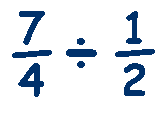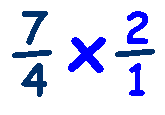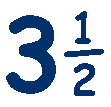# Dividing Fractions

To divide fractions, we convert the calculation from a divide to multiplication
and then treat it as a multiplication.

First though we need to change any mixed numbers into improper fractions.

Next we turn the second fraction upside down and multiply them as before.

Lets look at an example:Change any mixed numbers into improper fractionsFlip the second fraction and change divide to multiplyMultiply as before and simplifyor cancel outLastly, convert from an improper fraction to a proper fraction, to giveTry this

Fraction Division Worksheet

and

Dividing Fractions Quiz

from Dr. Mike